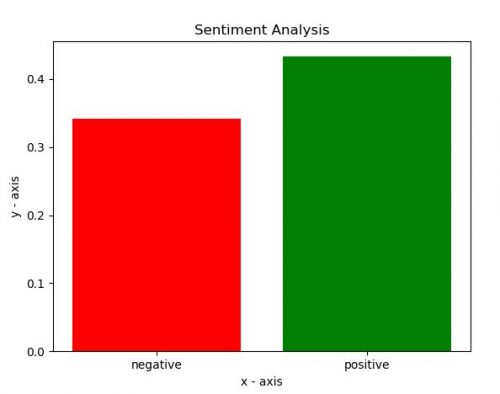Sentiment analysis (also known as opinion mining ) refers to the use of natural language processing, text analysis, computational linguistics to systematically identify, extract, quantify, and study affective states and subjective information.  In short, Sentiment analysis gives an objective idea of whether the text uses mostly positive, negative, or neutral language. 

Sentiment analysis software can assist estimate people opinion on the events in finance world, generate reports for relevant information, analyze correlation between events and stock prices.Image by Gino Crescoli from Pixabay

## The problem

In this post we investigate how to extract information about company and detect its sentiment. For each text sentence or paragraph we will detect its positivity or negativity by calculating sentiment score. This also called polarity. Polarity in sentiment analysis refers to identifying sentiment orientation (positive, neutral, and negative) in the text.

Given the list of companies we want to find polarity of sentiment in the text that has names of companies from the list. Below is the description how it can be implemented.

## Getting Data

We will use google to collect data. For this we search google via script for documents with some predefined keywords.
It will return the links that we will save to array.

```try:
except ImportError:

# to search
query = "financial_news Warren Buffett 2019"

for j in search(query, tld="co.in", num=10, stop=10, pause=6):
print(j)
```

## Preprocessing

After we got links, we need get text documents and remove not needed text and characters. So in this step we remove html tags, not valid characters. We keep however paragraph tags. Using paragraph tag we divide text document in smaller text units. After that we remove p tags.

```para_data=[]

def get_text(url):
print (url)

try:
req  = requests.get(url, timeout=5)
except:
return "TIMEOUT ERROR"

data = req.text
soup = BeautifulSoup(data, "html.parser")

paras=[]
paras_ = soup.find_all('p')
filtered_paras= filter(tag_visible, paras_)
for s in filtered_paras:
paras.append(s)
if len(paras) > 0:
for i, para in enumerate(paras):
para=remove_tags(para)
# remove non text characters
para_data.append(clean_txt(para))
```

## Calculating Sentiment

Now we calculate sentiment score using VADER (Valence Aware Dictionary and sEntiment Reasoner) VADER is a lexicon and rule-based sentiment analysis tool that is specifically attuned to sentiments. Based on calculated sentiment we build plot. In this example we only build plot for first company name which is Coca Cola.```from vaderSentiment.vaderSentiment import SentimentIntensityAnalyzer

def sentiment_scores(sentence):

# Create a SentimentIntensityAnalyzer object.
sid_obj = SentimentIntensityAnalyzer()

# polarity_scores method of SentimentIntensityAnalyzer
# oject gives a sentiment dictionary.
# which contains pos, neg, neu, and compound scores.
sentiment_dict = sid_obj.polarity_scores(sentence)

print("Overall sentiment dictionary is : ", sentiment_dict)
print("sentence was rated as ", sentiment_dict['neg']*100, "% Negative")
print("sentence was rated as ", sentiment_dict['neu']*100, "% Neutral")
print("sentence was rated as ", sentiment_dict['pos']*100, "% Positive")

# decide sentiment as positive, negative and neutral
if sentiment_dict['compound'] >= 0.05 :
print("Positive")

elif sentiment_dict['compound'] <= - 0.05 :
print("Negative")

else :
print("Neutral")
return sentiment_dict['compound']
```

Below you can find full source code.

```# -*- coding: utf-8 -*-
from bs4 import BeautifulSoup, NavigableString
from bs4.element import Comment
import requests
import re

TAG_RE = re.compile(r'<[^>]+>')

def remove_tags(text_string):
print (text_string)

return TAG_RE.sub('', str(text_string))

MIN_LENGTH_of_document = 40
MIN_LENGTH_of_word = 2

# function to print sentiments
# of the sentence.
# below function based on function from https://www.geeksforgeeks.org/python-sentiment-analysis-using-vader/
def sentiment_scores(sentence):

# Create a SentimentIntensityAnalyzer object.
sid_obj = SentimentIntensityAnalyzer()

# polarity_scores method of SentimentIntensityAnalyzer
# oject gives a sentiment dictionary.
# which contains pos, neg, neu, and compound scores.
sentiment_dict = sid_obj.polarity_scores(sentence)

print("Overall sentiment dictionary is : ", sentiment_dict)
print("sentence was rated as ", sentiment_dict['neg']*100, "% Negative")
print("sentence was rated as ", sentiment_dict['neu']*100, "% Neutral")
print("sentence was rated as ", sentiment_dict['pos']*100, "% Positive")
print("Sentence Overall Rated As", end = " ")

return sentiment_dict['compound']

def remove_min_words(txt):
# https://www.w3resource.com/python-exercises/re/python-re-exercise-49.php
shortword = re.compile(r'\W*\b\w{1,1}\b')
return(shortword.sub('', txt))

def clean_txt(text):

text = re.sub('[^A-Za-z.  ]', ' ', str(text))
text=' '.join(text.split())
text = remove_min_words(text)
text=text.lower()
text = text if  len(text) >= MIN_LENGTH_of_document else ""
return text

def between(cur, end):
while cur and cur != end:
if isinstance(cur, NavigableString):
text = cur.strip()
if len(text):
yield text
cur = cur.next_element

def next_element(elem):
while elem is not None:
# Find next element, skip NavigableString objects
elem = elem.next_sibling
if hasattr(elem, 'name'):
return elem

def tag_visible(element):
if element.parent.name in ['style', 'script', 'head',  'meta', '[document]']:
return False
if isinstance(element, Comment):
return False
return True

para_data=[]

def get_text(url):
print (url)

try:
req  = requests.get(url, timeout=5)
except:
return "TIMEOUT ERROR"

data = req.text
soup = BeautifulSoup(data, "html.parser")

paras=[]
paras_ = soup.find_all('p')
filtered_paras= filter(tag_visible, paras_)
for s in filtered_paras:
paras.append(s)
if len(paras) > 0:
for i, para in enumerate(paras):
para=remove_tags(para)
# remove non text characters
para_data.append(clean_txt(para))

try:
except ImportError:

# to search
query = "coca cola 2019"

for j in search(query, tld="co.in", num=25, stop=25, pause=6):
print(j)

# Here our list consists from one company name, but it can include more than one.
orgs=["coca cola" ]

results=[]
count=0

def update_dict_value( dict, key, value):
if key in dict:
dict[key]= dict[key]+value
else:
dict[key] =value
return dict

# will update paras - array of paragraphs

for pr in para_data:

for org in orgs:
if pr.find (org) >=0:
# extract sentiment
score=0
results.append ([org, sentiment_scores(pr), pr])

positive={}
negative={}
positive_sentiment={}
negative_sentiment={}

for i in range(len(results)):
org = results[i]

if (results[i] >=0):
positive = update_dict_value( positive, org, 1)
positive_sentiment =  update_dict_value( positive_sentiment, org,results[i])

else:
negative = update_dict_value( negative, org, 1)
negative_sentiment =  update_dict_value( negative_sentiment, org,results[i])

for org in orgs:

positive_sentiment[org]=positive_sentiment[org] / positive[org]
print (negative_sentiment[org])
negative_sentiment[org]=negative_sentiment[org] / negative[org]

import matplotlib.pyplot as plt

# x-coordinates of left sides of bars
labels = ['negative', 'positive']

# heights of bars
sentiment = [(-1)*negative_sentiment[orgs], positive_sentiment[orgs]]

# labels for bars
tick_label = ['negative', 'positive']

# plotting a bar chart
plt.bar(labels, sentiment, tick_label = tick_label,
width = 0.8, color = ['red', 'green'])

# naming the x-axis
plt.xlabel('x - axis')
# naming the y-axis
plt.ylabel('y - axis')
# plot title
plt.title('Sentiment Analysis')

# function to show the plot
plt.show()
```

References
1. Sentiment analysis Wikipedia
2. What is a “Sentiment Score” and how is it measured?